Intermediate Algebra

# 11.2Parabolas

Intermediate Algebra11.2 Parabolas

### Learning Objectives

By the end of this section, you will be able to:
• Graph vertical parabolas
• Graph horizontal parabolas
• Solve applications with parabolas

### Be Prepared 11.2

Before you get started, take this readiness quiz.

1. Graph: $y=−3x2+12x−12.y=−3x2+12x−12.$
If you missed this problem, review Example 9.47.
2. Solve by completing the square: $x2−6x+6=0.x2−6x+6=0.$
If you missed this problem, review Example 9.12.
3. Write in standard form: $y=3x2−6x+5.y=3x2−6x+5.$
If you missed this problem, review Example 9.59.

### Graph Vertical Parabolas

The next conic section we will look at is a parabola. We define a parabola as all points in a plane that are the same distance from a fixed point and a fixed line. The fixed point is called the focus, and the fixed line is called the directrix of the parabola.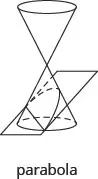### Parabola

A parabola is all points in a plane that are the same distance from a fixed point and a fixed line. The fixed point is called the focus, and the fixed line is called the directrix of the parabola.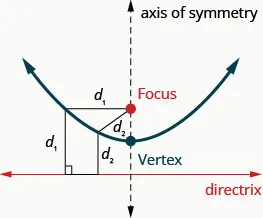Previously, we learned to graph vertical parabolas from the general form or the standard form using properties. Those methods will also work here. We will summarize the properties here.

Vertical Parabolas
General form
$y=ax2+bx+cy=ax2+bx+c$
Standard form
$y=a(x−h)2+ky=a(x−h)2+k$
Orientation $a>0a>0$ up; $a<0a<0$ down $a>0a>0$ up; $a<0a<0$ down
Axis of symmetry $x=−b2ax=−b2a$ $x=hx=h$
Vertex Substitute $x=−b2ax=−b2a$ and
solve for y.
$(h,k)(h,k)$
y-intercept Let $x=0x=0$ Let $x=0x=0$
x-intercepts Let $y=0y=0$ Let $y=0y=0$

The graphs show what the parabolas look like when they open up or down. Their position in relation to the x- or y-axis is merely an example.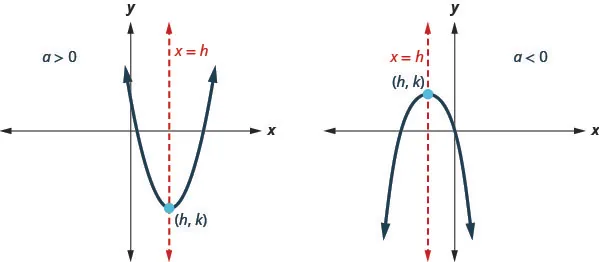To graph a parabola from these forms, we used the following steps.

### How To

#### Graph vertical parabolas $(y=ax2+bx+corf(x)=a(x−h)2+k)(y=ax2+bx+corf(x)=a(x−h)2+k)$ using properties.

1. Step 1. Determine whether the parabola opens upward or downward.
2. Step 2. Find the axis of symmetry.
3. Step 3. Find the vertex.
4. Step 4. Find the y-intercept. Find the point symmetric to the y-intercept across the axis of symmetry.
5. Step 5. Find the x-intercepts.
6. Step 6. Graph the parabola.

The next example reviews the method of graphing a parabola from the general form of its equation.

### Example 11.12

Graph $y=−x2+6x−8y=−x2+6x−8$ by using properties.

### Try It 11.23

Graph $y=−x2+5x−6y=−x2+5x−6$ by using properties.

### Try It 11.24

Graph $y=−x2+8x−12y=−x2+8x−12$ by using properties.

The next example reviews the method of graphing a parabola from the standard form of its equation, $y=a(x−h)2+k.y=a(x−h)2+k.$

### Example 11.13

Write$y=3x2−6x+5y=3x2−6x+5$ in standard form and then use properties of standard form to graph the equation.

### Try It 11.25

Write $y=2x2+4x+5y=2x2+4x+5$ in standard form and use properties of standard form to graph the equation.

### Try It 11.26

Write $y=−2x2+8x−7y=−2x2+8x−7$ in standard form and use properties of standard form to graph the equation.

### Graph Horizontal Parabolas

Our work so far has only dealt with parabolas that open up or down. We are now going to look at horizontal parabolas. These parabolas open either to the left or to the right. If we interchange the x and y in our previous equations for parabolas, we get the equations for the parabolas that open to the left or to the right.

Horizontal Parabolas
General form
$x=ay2+by+cx=ay2+by+c$
Standard form
$x=a(y−k)2+hx=a(y−k)2+h$
Orientation $a>0a>0$ right; $a<0a<0$ left $a>0a>0$ right; $a<0a<0$ left
Axis of symmetry $y=−b2ay=−b2a$ $y=ky=k$
Vertex Substitute $y=−b2ay=−b2a$ and
solve for x.
$(h,k)(h,k)$
y-intercepts Let $x=0x=0$ Let $x=0x=0$
x-intercept Let $y=0y=0$ Let $y=0y=0$
Table 11.1

The graphs show what the parabolas look like when they to the left or to the right. Their position in relation to the x- or y-axis is merely an example.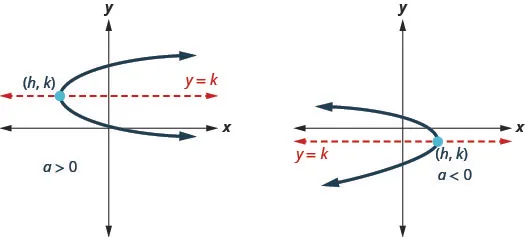Looking at these parabolas, do their graphs represent a function? Since both graphs would fail the vertical line test, they do not represent a function.

To graph a parabola that opens to the left or to the right is basically the same as what we did for parabolas that open up or down, with the reversal of the x and y variables.

### How To

#### Graph horizontal parabolas $(x=ay2+by+corx=a(y−k)2+h)(x=ay2+by+corx=a(y−k)2+h)$ using properties.

1. Step 1. Determine whether the parabola opens to the left or to the right.
2. Step 2. Find the axis of symmetry.
3. Step 3. Find the vertex.
4. Step 4. Find the x-intercept. Find the point symmetric to the x-intercept across the axis of symmetry.
5. Step 5. Find the y-intercepts.
6. Step 6. Graph the parabola.

### Example 11.14

Graph $x=2y2x=2y2$ by using properties.

### Try It 11.27

Graph $x=y2x=y2$ by using properties.

### Try It 11.28

Graph $x=−y2x=−y2$ by using properties.

In the next example, the vertex is not the origin.

### Example 11.15

Graph $x=−y2+2y+8x=−y2+2y+8$ by using properties.

### Try It 11.29

Graph $x=−y2−4y+12x=−y2−4y+12$ by using properties.

### Try It 11.30

Graph $x=−y2+2y−3x=−y2+2y−3$ by using properties.

In Table 11.1, we see the relationship between the equation in standard form and the properties of the parabola. The How To box lists the steps for graphing a parabola in the standard form $x=a(y−k)2+h.x=a(y−k)2+h.$ We will use this procedure in the next example.

### Example 11.16

Graph $x=2(y−2)2+1x=2(y−2)2+1$ using properties.

### Try It 11.31

Graph $x=3(y−1)2+2x=3(y−1)2+2$ using properties.

### Try It 11.32

Graph $x=2(y−3)2+2x=2(y−3)2+2$ using properties.

In the next example, we notice the a is negative and so the parabola opens to the left.

### Example 11.17

Graph $x=−4(y+1)2+4x=−4(y+1)2+4$ using properties.

### Try It 11.33

Graph $x=−4(y+2)2+4x=−4(y+2)2+4$ using properties.

### Try It 11.34

Graph $x=−2(y+3)2+2x=−2(y+3)2+2$ using properties.

The next example requires that we first put the equation in standard form and then use the properties.

### Example 11.18

Write $x=2y2+12y+17x=2y2+12y+17$ in standard form and then use the properties of the standard form to graph the equation.

### Try It 11.35

Write $x=3y2+6y+7x=3y2+6y+7$ in standard form and use properties of the standard form to graph the equation.

### Try It 11.36

Write $x=−4y2−16y−12x=−4y2−16y−12$ in standard form and use properties of the standard form to graph the equation.

### Solve Applications with Parabolas

Many architectural designs incorporate parabolas. It is not uncommon for bridges to be constructed using parabolas as we will see in the next example.

### Example 11.19

Find the equation of the parabolic arch formed in the foundation of the bridge shown. Write the equation in standard form.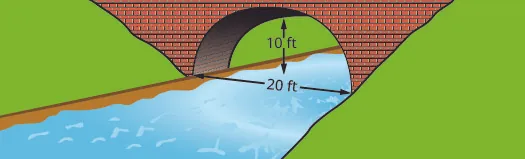### Try It 11.37

Find the equation of the parabolic arch formed in the foundation of the bridge shown. Write the equation in standard form.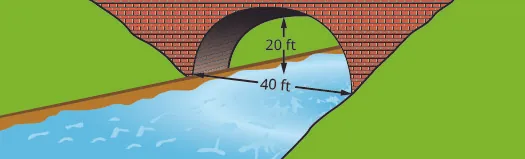### Try It 11.38

Find the equation of the parabolic arch formed in the foundation of the bridge shown. Write the equation in standard form.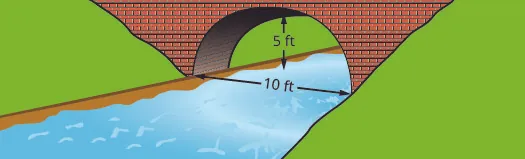### Media

Access these online resources for additional instructions and practice with quadratic functions and parabolas.

### Section 11.2 Exercises

#### Practice Makes Perfect

Graph Vertical Parabolas

In the following exercises, graph each equation by using properties.

53.

$y = − x 2 + 4 x − 3 y = − x 2 + 4 x − 3$

54.

$y = − x 2 + 8 x − 15 y = − x 2 + 8 x − 15$

55.

$y = 6 x 2 + 2 x − 1 y = 6 x 2 + 2 x − 1$

56.

$y = 8 x 2 − 10 x + 3 y = 8 x 2 − 10 x + 3$

In the following exercises, write the equation in standard form and use properties of the standard form to graph the equation.

57.

$y = − x 2 + 2 x − 4 y = − x 2 + 2 x − 4$

58.

$y = 2 x 2 + 4 x + 6 y = 2 x 2 + 4 x + 6$

59.

$y = −2 x 2 − 4 x − 5 y = −2 x 2 − 4 x − 5$

60.

$y = 3 x 2 − 12 x + 7 y = 3 x 2 − 12 x + 7$

Graph Horizontal Parabolas

In the following exercises, graph each equation by using properties.

61.

$x = −2 y 2 x = −2 y 2$

62.

$x = 3 y 2 x = 3 y 2$

63.

$x = 4 y 2 x = 4 y 2$

64.

$x = −4 y 2 x = −4 y 2$

65.

$x = − y 2 − 2 y + 3 x = − y 2 − 2 y + 3$

66.

$x = − y 2 − 4 y + 5 x = − y 2 − 4 y + 5$

67.

$x = y 2 + 6 y + 8 x = y 2 + 6 y + 8$

68.

$x = y 2 − 4 y − 12 x = y 2 − 4 y − 12$

69.

$x = ( y − 2 ) 2 + 3 x = ( y − 2 ) 2 + 3$

70.

$x = ( y − 1 ) 2 + 4 x = ( y − 1 ) 2 + 4$

71.

$x = − ( y − 1 ) 2 + 2 x = − ( y − 1 ) 2 + 2$

72.

$x = − ( y − 4 ) 2 + 3 x = − ( y − 4 ) 2 + 3$

73.

$x = ( y + 2 ) 2 + 1 x = ( y + 2 ) 2 + 1$

74.

$x = ( y + 1 ) 2 + 2 x = ( y + 1 ) 2 + 2$

75.

$x = − ( y + 3 ) 2 + 2 x = − ( y + 3 ) 2 + 2$

76.

$x = − ( y + 4 ) 2 + 3 x = − ( y + 4 ) 2 + 3$

77.

$x = −3 ( y − 2 ) 2 + 3 x = −3 ( y − 2 ) 2 + 3$

78.

$x = −2 ( y − 1 ) 2 + 2 x = −2 ( y − 1 ) 2 + 2$

79.

$x = 4 ( y + 1 ) 2 − 4 x = 4 ( y + 1 ) 2 − 4$

80.

$x = 2 ( y + 4 ) 2 − 2 x = 2 ( y + 4 ) 2 − 2$

In the following exercises, write the equation in standard form and use properties of the standard form to graph the equation.

81.

$x = y 2 + 4 y − 5 x = y 2 + 4 y − 5$

82.

$x = y 2 + 2 y − 3 x = y 2 + 2 y − 3$

83.

$x = −2 y 2 − 12 y − 16 x = −2 y 2 − 12 y − 16$

84.

$x = −3 y 2 − 6 y − 5 x = −3 y 2 − 6 y − 5$

Mixed Practice

In the following exercises, match each graph to one of the following equations: x2 + y2 = 64 x2 + y2 = 49
(x + 5)2 + (y + 2)2 = 4 (x − 2)2 + (y − 3)2 = 9 y = −x2 + 8x − 15 y = 6x2 + 2x − 1

85.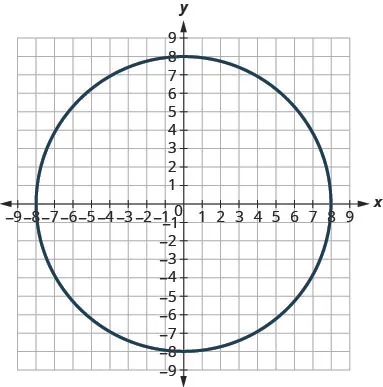86.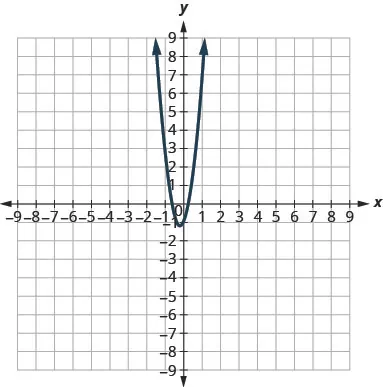87.88.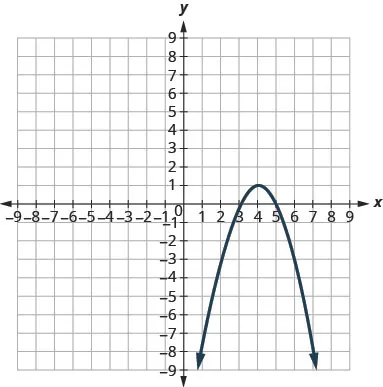89.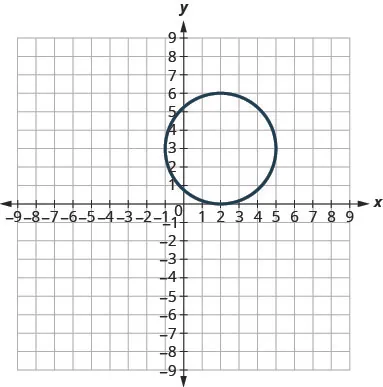90.Solve Applications with Parabolas

91.

Write the equation in standard form of the parabolic arch formed in the foundation of the bridge shown. Use the lower left side of the bridge as the origin (0, 0).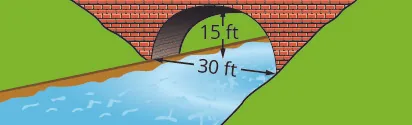92.

Write the equation in standard form of the parabolic arch formed in the foundation of the bridge shown. Use the lower left side of the bridge as the origin (0, 0).93.

Write the equation in standard form of the parabolic arch formed in the foundation of the bridge shown. Use the lower left side of the bridge as the origin (0, 0).94.

Write the equation in standard form of the parabolic arch formed in the foundation of the bridge shown. Use the lower left side of the bridge as the origin (0, 0).#### Writing Exercises

95.

In your own words, define a parabola.

96.

Is the parabola $y=x2y=x2$ a function? Is the parabola $x=y2x=y2$ a function? Explain why or why not.

97.

Write the equation of a parabola that opens up or down in standard form and the equation of a parabola that opens left or right in standard form. Provide a sketch of the parabola for each one, label the vertex and axis of symmetry.

98.

Explain in your own words, how you can tell from its equation whether a parabola opens up, down, left or right.

#### Self Check

After completing the exercises, use this checklist to evaluate your mastery of the objectives of this section.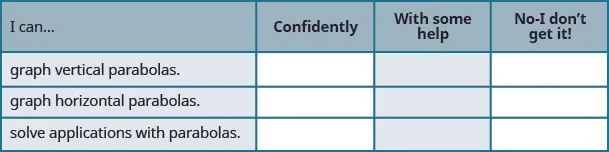After reviewing this checklist, what will you do to become confident for all objectives?

Order a print copy

As an Amazon Associate we earn from qualifying purchases.